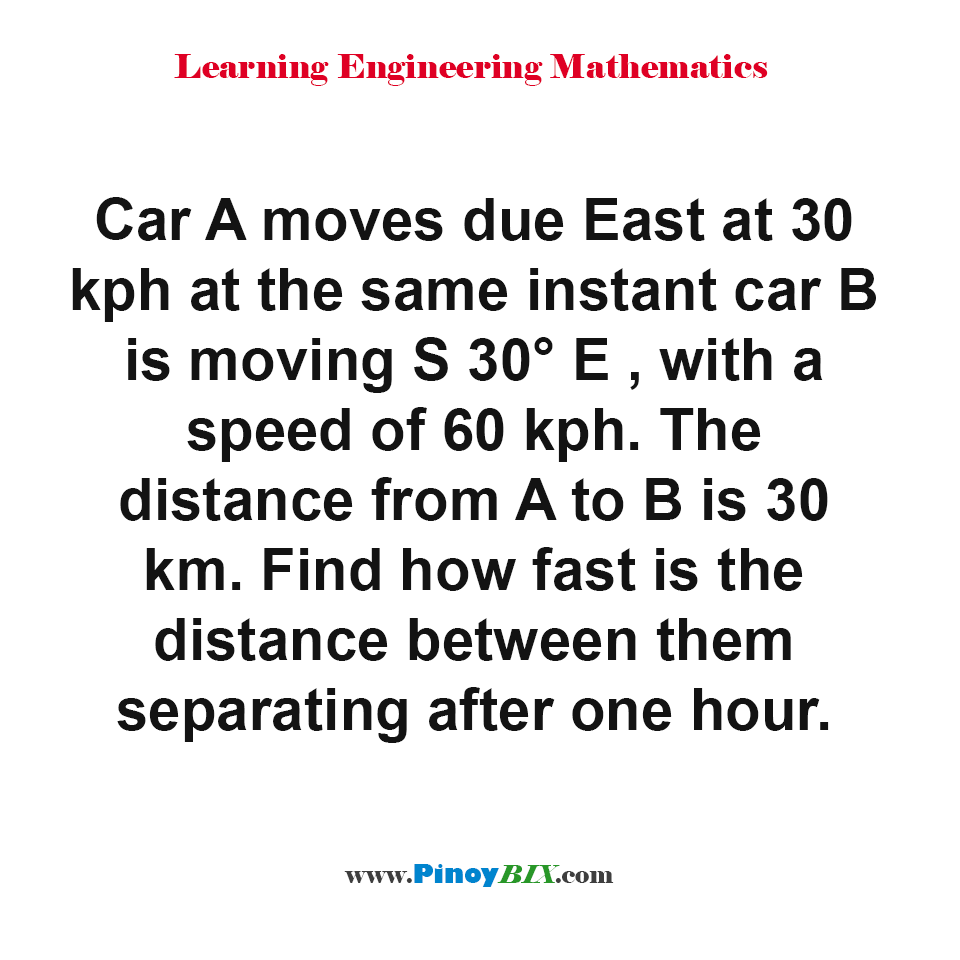# Solution: How fast is the distance between car A and car B separating after one hour?

(Last Updated On: January 21, 2020)#### Problem Statement: CE Board May 1997

Car A moves due East at 30 kph at the same instant car B is moving S 30° E , with a speed of 60 kph. The distance from A to B is 30 km. Find how fast is the distance between them separating after one hour.

• A. 36 kph
• B. 38 kph
• C. 40 kph
• D. 45 kph

The distance between car A and car B separating at the rate of 45 kph

#### Latest Problem Solving in Differential Calculus (MAXIMA-MINIMA & TIME RATES)

More Questions in: Differential Calculus (MAXIMA-MINIMA & TIME RATES)